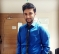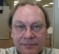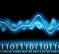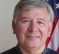## Standard deviation in DSPStarted by 6 years ago10 replieslatest reply 6 years ago676 views

i've started reading dspguide.com and came across through this.

This method of calculating the mean and standard deviation is adequate for many applications; however, it has two limitations. First, if the mean is much larger than the standard deviation, Eq. 2-2 involves subtracting two numbers that are very close in value. This can result in excessive round-off error in the calculations. Second, it is often desirable to recalculate the mean and standard deviation as new samples are acquired and added to the signal. We will call this type of calculation: running statistics. While the method of Eqs. 2-1 and 2-2 can be used for running statistics, it requires that all of the samples be involved in each new calculation. This is a very inefficient use of computational power and memory.how did the author conclude that if mean is larger than standard deviation then x[i] and μ are very close.

and how does the round-off error occur in the calculations?

[ - ]>and how does the round-off error occur in the calculations?

>how did the author conclude that if mean is larger than standard deviation then x[i] and μ are very close.

This is one of the properties of mean and standard deviation; if you calculate $$k_i = \dfrac{x_i - \mu}{\sigma}$$ then a very large fraction of $$k_i$$ have $$|k_i| < 6$$ (in other words, the vast majority of data points are within 6 standard deviations of the mean).

edit: regarding round-off and this specific calculation, your best bet is to read Knuth's The Art of Computer Programming vol 2, which talks about Welford's algorithm that I discussed in one of my articles.

[ - ]You mean $$\left| k_i \right| < 6\sigma$$.  Otherwise -- yes, you beat me to it, and said exactly what I would have said.

[ - ]No, what I posted was correct; $$k_i$$ is normalized to represent the number of standard deviations away from the mean.

[ - ]Whoops.  I missed that.  Sorry.

[ - ]>and how does the round-off error occur in the calculations?

jms_nh's link answers this, but the short answer is that floating-point numbers are subject to the "subtracting two big numbers to get a little number is inaccurate" rule.  What constitutes "big" and "little" and "inaccurate" are subject to the problem at hand, so it's good to understand the underlying math -- which, I trust, is in the referenced paper.

[ - ]The way I visualise this issue (for electronic signals at least) is that the mean is the "dc" offset while standard of deviation is the "AC" mean.

Thus if mean (i.e. DC offset) is very high it will waste a lot of bit resolution at implementation.

The mean can be subtracted first to remove dc offset. Then all resolution becomes available for AC mean. I wonder if it is useful then to add the DC offset back.

[ - ]However, DC can be an important signal component. If the signal under test is a direct demodulated version of an RF signal, then for AM modulated signals (e.g. ILS, VOR, and similar), the DC represents the carrier level (essentially the inverse of the generating function). If the demodulation is done in a receiver with AGC, then the DC represents the receiver's AGC reference level and is not related to the carrier level, so "extracting" the carrier's level must be done by calculating the AGC gain based on the receiver's transfer function or other means related to the receiver design (e.g. log amp).

Note that when the signal's DC representing carrier level is a critical signal component, then any real DC offsets are errors and must be estimated and removed to determine the true RF carrier level. In that case, each signal component should be processed separately for stddev and not as an ensemble or group (think apples and oranges). In fact, for an ILS (Instrument Landing System) signal, the difference in the 90 and 150 Hz AM components represents a plane's azimuth (horizontal) or glidepath (vertical) position relative to the runway's centerline and touchdown point, respectively. So in that case, errors in the 90 and 150 Hz components must be estimated separately.

[ - ]>how did the author conclude that if mean is larger than standard deviation then x[i] and μ are very close.

σ^2 = E{(x - μ)^2}, where E{x} = μ.

μ >> σ^2, for example μ > 10*σ^2 =>  E{(x - μ)^2} < μ/10.

The relationship   E{(x - μ)^2} < μ/10 suggests that the difference

(x - μ)^2  has   μ/10 as a superior bound.

[ - ]You ask, how did the author reach this conclusion:

First, if the mean is much larger than the standard deviation, Eq. 2-2 involves subtracting two numbers that are very close in value.

You start with a large mean value and a small standard deviation.  Picturing this, the x[i] must all be near the mean so they are both large numbers and nearly equal.  So, the difference is going to be subject to errors.  That's all....

Now, you may want to ask: Why would the difference be subject to errors and that's the point that jms_nh has made I believe.

[ - ]